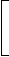# Data Interpretation - Pie Charts

Directions to Solve

Study the following pie-diagrams carefully and answer the questions given below it:

Percentage Composition of Human Body1.
What percent of the total weight of human body is equivalent to the weight of the proteins in skin in human body?
0.016
1.6
0.16
Explanation:

Let the body weight be x kg.

Then, weight of skin protein in the body
 =16% of1 of xkg 10
 =16 xkg. 1000Required percentage =16x1000
x 100% = 1.6%.
x

2.
What will be the quantity of water in the body of a person weighing 50 kg?
20 kg
35 kg
41 kg
42.5 kg
Explanation:

 Quantity of water in the body of a person weighing 50 kg = (70% of 50) kg = 35 kg.

3.
What is the ratio of the distribution of proteins in the muscles to that of the distribution of proteins in the bones?
1 : 18
1 : 2
2 : 1
18 : 1
Explanation:

Required ratio =
 16% of 1 3
= 6 = 2 .
 16% of 1 6
3 1

4.
To show the distribution of proteins and other dry elements in the human body, the arc of the circle should subtend at the centre an angle of:
54°
126°
108°
252°
Explanation:

 Percentage of proteins and other dry elements in the body = (16% + 14%) = 30%.Central angle corresponding to proteins and other dry elements together = 30% of 360° = 108°.

5.
In the human body, what part is made of neither bones nor skin?
 1 40
 3 80
 2 5
None of these
 Part of the body made of neither bones nor skin = 1 -1 + 1= 11 . 6 10 15